# Fibonacci Series using Recursion in C

## Fibonacci Series using Recursion in C with Examples

In this article, I am going to discuss Fibonacci Series using Recursion in C Language with Examples. Please read our previous article, where we discussed the Combination Formula (NCR) using Recursion in C Language with Examples. Here, first, we will discuss what the Fibonacci series is, then we will implement the Fibonacci series program using Iteration, and finally, we will implement the Fibonacci series program using Recursion in C language with Examples along with the Tracing tree.

##### What is Fibonacci Series?

First, let us understand what Fibonacci Series is, and then we will create a recursive definition, and then we will analyze that function. Below is the sequence of the Fibonacci Series: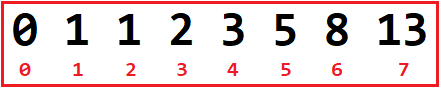In the above diagram, the 0th term is 0, and 1st term is 1 and after it, the next term is obtained by adding the previous two terms. Let’s have a look at the below image to understand it clearly.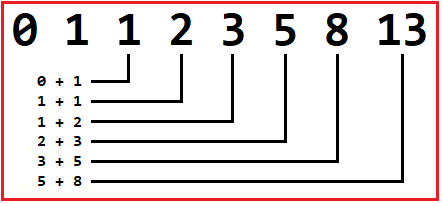As we can see in above Image,
0 + 1 = 1,
1 + 1 = 2,
1 + 2 = 3,
2 + 3 = 5,
And so on… So, this can be defined mathematically as,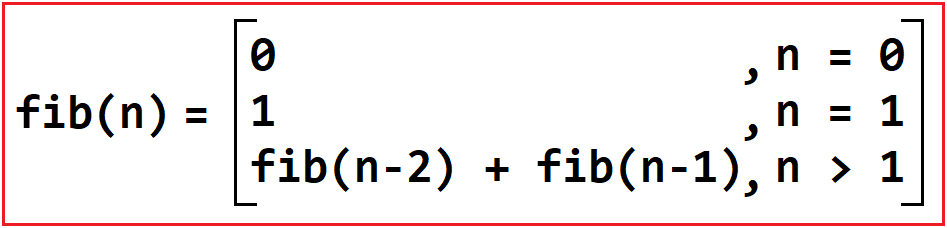So, now let’s write a recursive function for Fibonacci Series based on the above recurrence relation:

##### Fibonacci Series By using Loop in C Language:

This is the simplest approach and it will print the Fibonacci series by using the length. The following program shows how to use the iterative approach to print the Fibonacci Series in C up to a given length i.e. the number of elements to be printed in the Fibonacci series.

```#include <stdio.h>
void Fibonacci (int numberOfElements)
{
int firstNumber = 0, SecondNumber = 1, nextNumber;

//First print first and second number
printf ("%d %d", firstNumber, SecondNumber);

//Starts the loop from 2 because 0 and 1 are already printed
for (int i = 2; i < numberOfElements; i++)
{
nextNumber = firstNumber + SecondNumber;
printf (" %d", nextNumber);
firstNumber = SecondNumber;
SecondNumber = nextNumber;
}
}

int main ()
{
int numberOfElements;
printf("Enter the Length of the Fibonacci series: ");
scanf("%d", &numberOfElements);
if(numberOfElements < 2)
{
printf("Please Enter a number greater than two");
}
else
{
printf("The following is the Fibonacci series: ");
Fibonacci(numberOfElements);
}

return 0;
}
```
###### Output:

Enter the Length of the Fibonacci series: 10
The following is the Fibonacci series: 0 1 1 2 3 5 8 13 21 34

##### Recursive Approach to Print Fibonacci Series in C:

In the Recursive Approach, we need to pass the length of the Fibonacci Series to the recursive method and then it will iterate continuously until it reaches the goal. For better understanding, please have a look at the below example.

```#include <stdio.h>
void FibonacciSeries(int firstNumber, int secondNumber, int counter, int number)
{
printf ("%d ", firstNumber);
if (counter < number)
{
FibonacciSeries (secondNumber, firstNumber + secondNumber, counter + 1, number);
}
}

int main ()
{
int numberOfElements;
printf ("Enter the Length of the Fibonacci series: ");
scanf ("%d", &numberOfElements);
if (numberOfElements < 2)
{
printf ("Please Enter a number greater than two");
}
else
{
printf ("The following is the Fibonacci series: ");
FibonacciSeries (0, 1, 1, numberOfElements);
}
return 0;
}
```
###### Output:

Enter the Length of the Fibonacci series: 7
The following is the Fibonacci series: 0 1 1 2 3 5 8 7

Now let’s Jump on the Tracing Part.

#### Tracing Tree of the above Fibonacci Series Recursive Program:

##### Step1: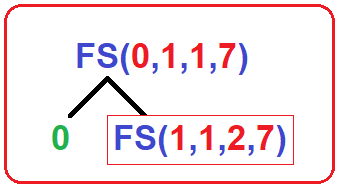Output: 0

Tree: Here, we are calling our FibonacciSeries function as FS(). In this method, we pass 4 parameters which are –

1. First number
2. Second number
3. counter
4. Number (nth term)

In the above Image, FS (0,1,1,7) is our 1st call. In this call, first we have to print first number which is 0 here, then it will check for if (1 < 7) i.e., (counter < number), Yes, then it will call itself as FS (1,1,2,7) with the following modified parameters:

1. First number = Second Number = 1
2. Second number = First Number + Second Number = 0 + 1 = 1
3. Counter = Counter + 1 = 1 + 1 = 2
4. Number will remain same for all recursive calls = 7
##### Step2: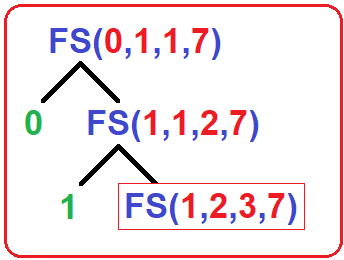Output: 0 1

Tree: Now, FS (1,1,2,7) is our 2nd call. It first prints the first number which is 1 here.

Then it will check for if (2 < 7) i.e., (counter < number), Yes, then it will call itself as FS (1,2,3,7) with the following modified parameters:

1. First number = Second Number = 1
2. Second number = First Number + Second Number = 1 + 1 = 2
3. Counter = Counter + 1 = 2 + 1 = 3
4. Number will remain same for all recursive calls = 7
##### Step3: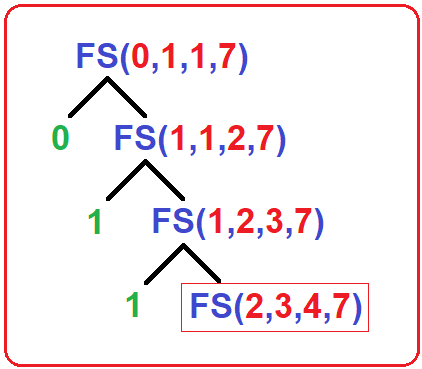Output: 0 1 1

Tree: Now, FS (1,2,3,7) is our 3rd call. It first prints the first number which is 1 here.

Then it will check for if (3 < 7) i.e., (counter < number), Yes, then it will call itself as FS (2,3,4,7) with the following modified parameters:

1. First number = Second Number = 2
2. Second number = First Number + Second Number = 1 + 2 = 3
3. Counter = Counter + 1 = 3 + 1 = 4
4. Number will remain same for all recursive calls = 7
##### Step4: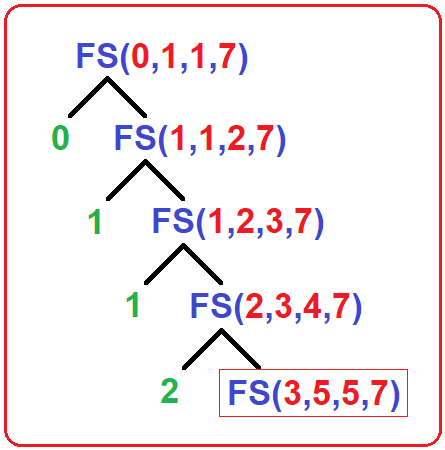Output: 0 1 1 2

Tree: Now, FS (2,3,4,7) is our 4th call. It first prints the first number which is 2 here.

Then it will check for if (4 < 7) i.e., (counter < number), Yes, then it will call itself as FS (3,5,5,7) with the following modified parameters:

1. First number = Second Number = 3
2. Second number = First Number + Second Number = 2 + 3 = 5
3. Counter = Counter + 1 = 4 + 1 = 5
4. Number will remain same for all recursive calls = 7
##### Step5: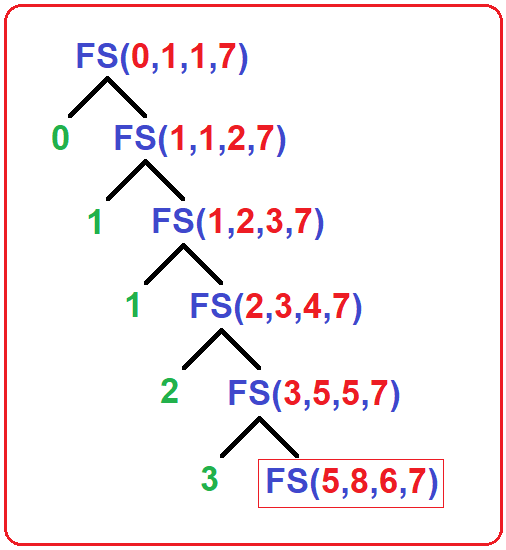Output: 0 1 1 2 3

Tree: Now, FS (3,5,5,7) is our 5th call. It first prints the first number which is 3 here.

Then it will check for if (5 < 7) i.e., (counter < number), Yes, then it will call itself as FS (5,8,6,7) with the following modified parameters:

1. First number = Second Number = 5
2. Second number = First Number + Second Number = 3 + 5 = 8
3. Counter = Counter + 1 = 5 + 1 = 6
4. Number will remain same for all recursive calls = 7
##### Step6: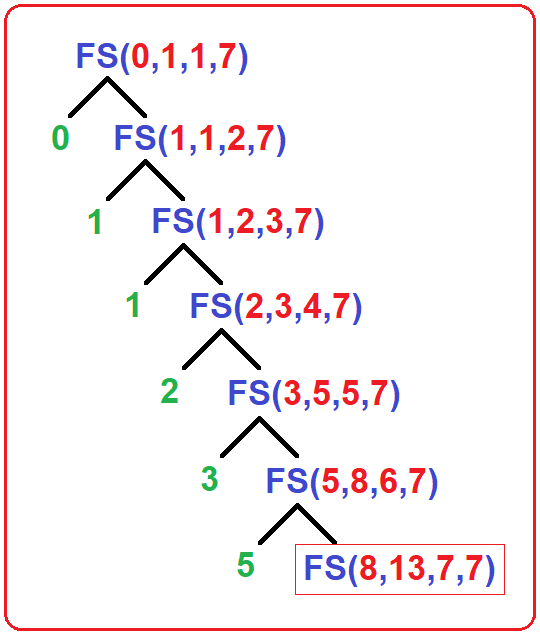Output: 0 1 1 2 3 5

Tree: Now, FS (5,8,6,7) is our 6th call. It first prints the first number which is 5 here.

Then it will check for if (6 < 7) i.e., (counter < number), Yes, then it will call itself as FS (8,13,7,7) with the following modified parameters:

1. First number = Second Number = 8
2. Second number = First Number + Second Number = 5 + 8 = 13
3. Counter = Counter + 1 = 6 + 1 = 7
4. Number will remain same for all recursive calls = 7
##### Step7: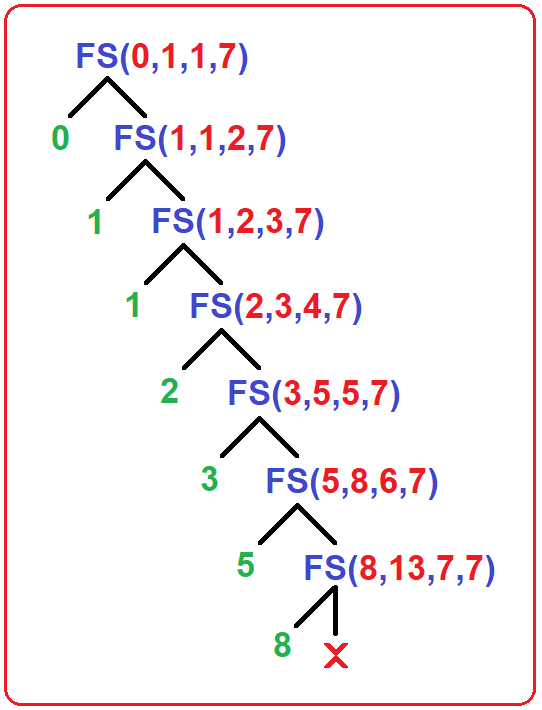Output: 0 1 1 2 3 5 8

Tree: Now, FS (8,13,7,7) is our 7th call. It first prints the first number which is 8 here. Then it will check for if (7 < 7) i.e., (counter < number), No, it will stop here and all the calls will finish here.

In the next article, I am going to discuss the Tower of Hanoi using Recursion in C Language with Examples. Here, in this article, I try to explain Fibonacci Series in C Language using Recursion with Example and I hope you enjoy this Fibonacci Series using Recursion in C Language with Example article.